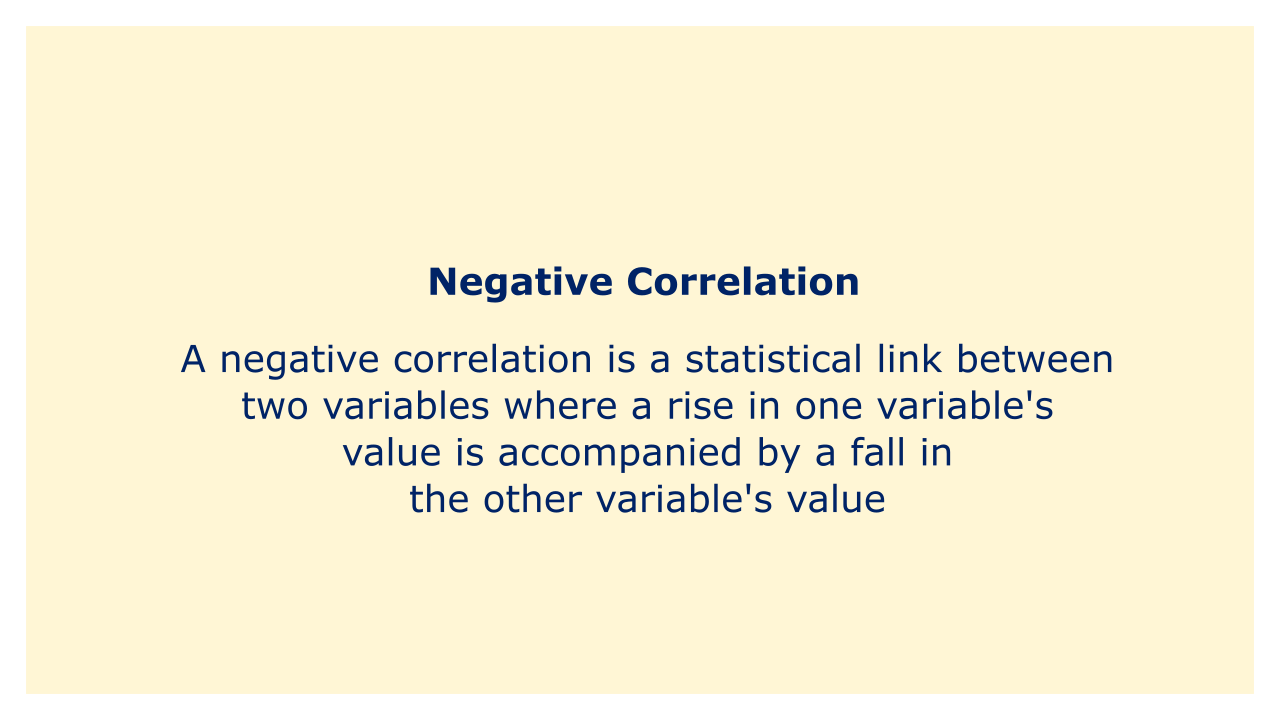# Negative CorrelationImage: Moneybestpal.com

### In the world of finance, a negative correlation is a statistical link between two variables where a rise in one variable's value is accompanied by a fall in the other variable's value.

To put it another way, when two variables have a negative correlation, they move in the opposite direction. Whenever one variable rises, the other one falls, and vice versa.

Think of two equities that are negatively connected as an example. Investors may switch their assets from risky equities to safer stocks during a slump in the stock market. As a result, the safer stock's value can rise while the riskier stock's value would decline. As a result, there is a negative correlation between the two stocks' valuations.

In financial portfolios, a negative correlation is frequently utilized to lower risk. Investors can diversify their portfolios and lessen their exposure to any one particular asset by investing in assets that have a negative correlation with one another. This is so that any losses from the value decline of one item are likely to be partially compensated by the value gain of the other asset.

A correlation coefficient is often used in finance to calculate the link between two variables. The correlation coefficient runs from -1 to 1, with a perfect negative correlation denoted by a value of -1, a perfect positive correlation by a value of 1, and no correlation denoted by a value of 0. A positive correlation coefficient shows that when one variable rises, the other one rises as well, whereas a negative correlation coefficient shows that when one variable rises, the other one rises as well.
Tags